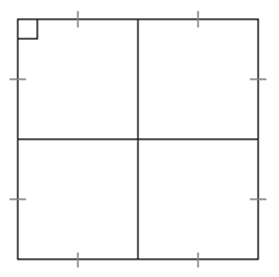# How Many Squares?

Directions: How many squares are shown in the diagram below?### Hint

What makes a square a square?

5 squares.  The interesting part about this problem is the vocabulary and parallelogram theorems involved in counting and proving what is a square.

Source:  This problem was found in the Fifth Edition Elementary Geometry for College Students by Alexander and Koeberlein

## Similar Triangles 2

Directions: Using the digits 0 to 9 at most one time each, create two similar …

1.••